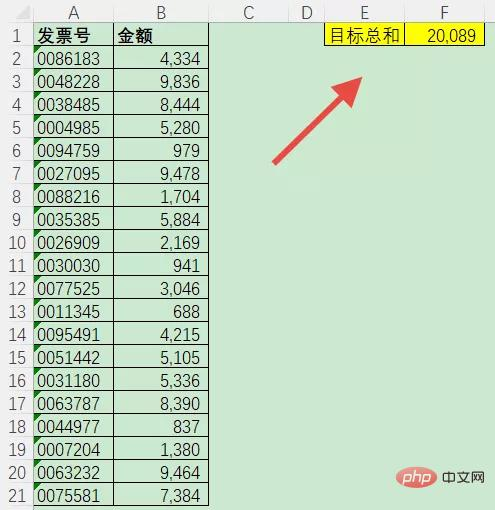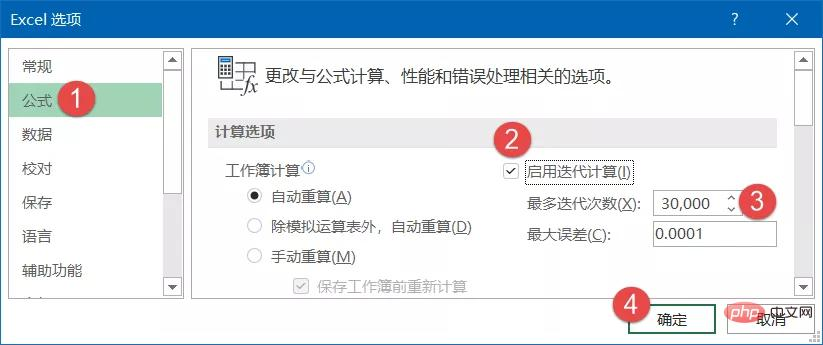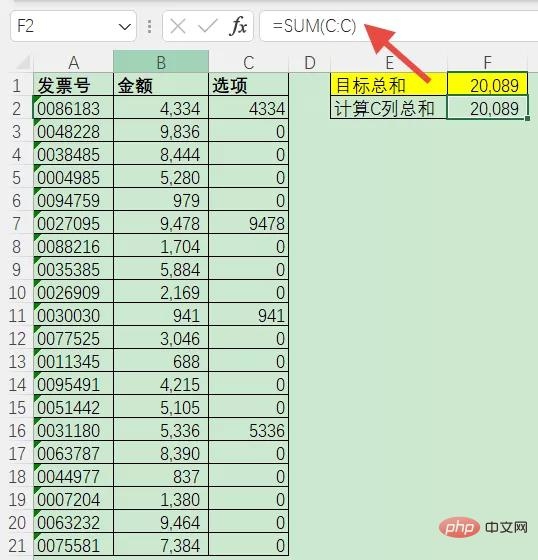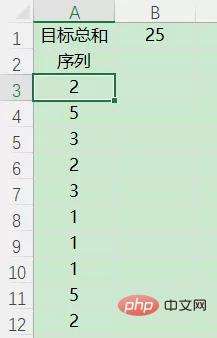﻿ 一起分析excel怎么使用函数寻找总和为某个值的组合 - 自由资讯

# 一起分析excel怎么使用函数寻找总和为某个值的组合`=IF(SUM(C\$2:C\$21)=F\$1,C2,B2*RANDBETWEEN(0,1))``=IF(SUM(C\$2:C\$21)=F\$1,C2,B2*RANDBETWEEN(0,1))`A3:A12输入公式

=IF(SUM(A\$3:A\$12)=B\$1,A3,RANDBETWEEN(1,5))﻿